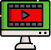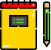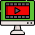VideosNotes

### Approximation

LOGARITHMS NUMBERS
Logarithm, the exponent or power to which a base must be raised to yield a given number.
Views: 1
Video Duration: 10:16
On the Same TopicLOGARITHMS
Logarithm, the exponent or ...Logarithmic Equations
i. Revision of logarithm of...Anti-Logarithm
The anti-logarithm of a num...Logarithm - Quotient Rule
Since eln(x/y)=eln(x)−ln(y)...
Other Related Videos